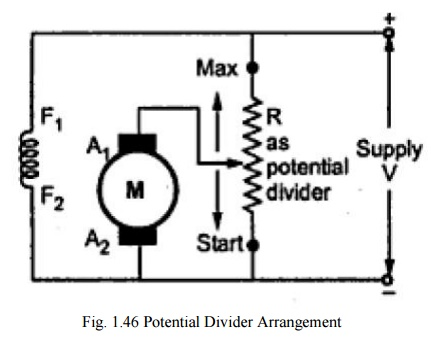Home | | Electrical Engineering and Instrumentation | Speed Control of DC Motors

# Speed Control of DC Motors

i. Flux control ii. Armature voltage control or rheostatic control iii. Applied voltage control iv. Potential divider control

Speed Control of DC Motors

## i. Flux controlAs speed is inversely proportional to the flux.

The flux is dependent on the current through the shunt field winding. Thus flux  can be controlled by adding a rheostat (variable resistance in series with the shunt field winding as shown in above figure.

At the beginning the rheostat is kept at minimum.

The supply voltage is at rated value. So current through the shunt field winding is  also its rated value. Hence the speed is also the rated value.

Resistance is increased, shunt field current is reduced (flux is reduced) and speed

is increased beyond its rated value.

## ii. Armature voltage control or rheostatic controlThe speed is directly proportional to the voltage applied across the armature.

As the supply voltage is normally constant, the voltage across the armature can be controlled by adding a variable resistance in series with the armature as shown in figure above.

## iii. Applied voltage controlShunt field of the motor is permanently connected to the fixed voltage supply.

Armature is supplied with various voltages by means of suitable switchgear arrangements.

## iv. Potential divider controlWhen the variable rheostat position is at start point shown, voltage across the armature is zero.

As rheostat is moved towards minimum point shown, the voltage across the armature increases, increasing the speed.

At maximum point the voltage is maximum and speed is rated value

Study Material, Lecturing Notes, Assignment, Reference, Wiki description explanation, brief detail
Electrical Engineering and Instrumentation : DC Machines : Speed Control of DC Motors |